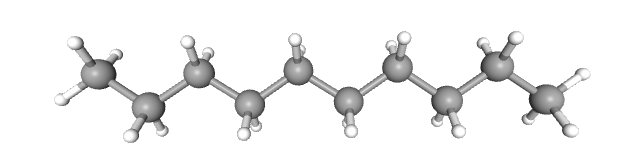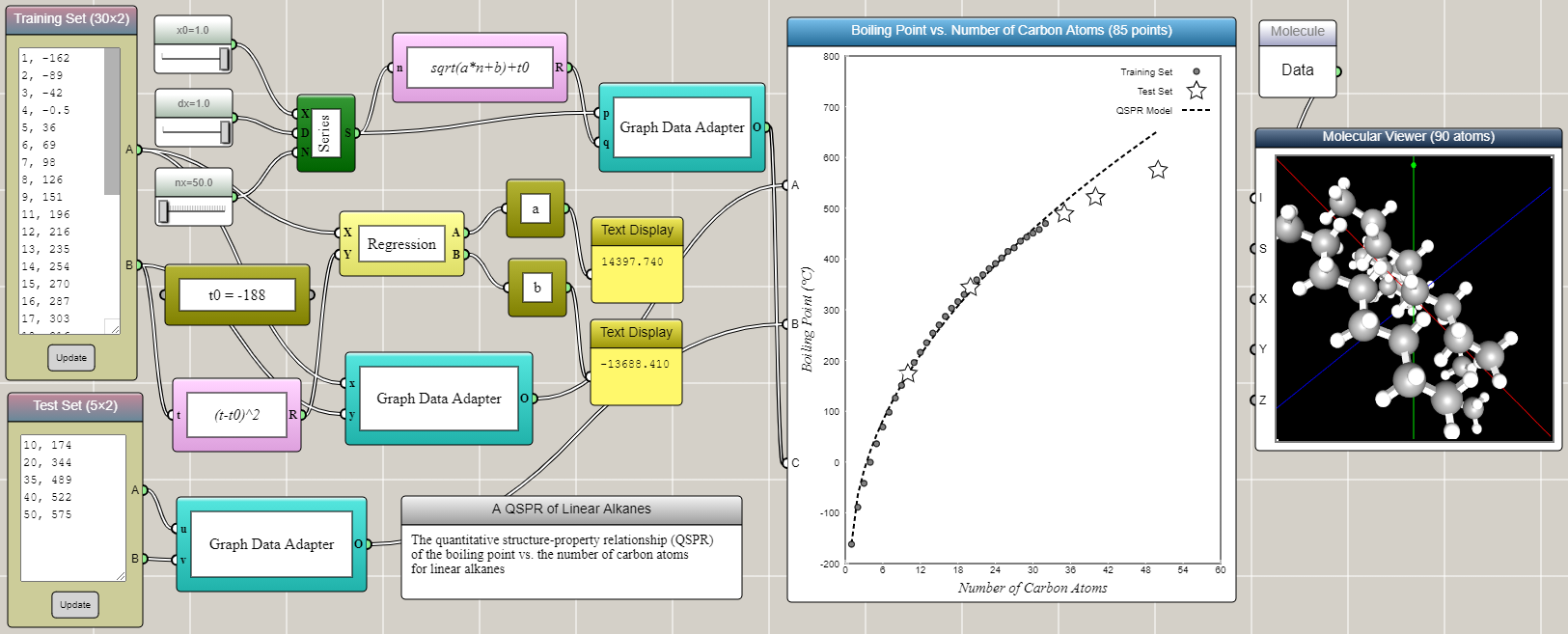#### Finding Quantitative Structure-Property Relationships using iFlow

By Charles Xie

In chemistry, quantitative structure–property relationship (QSPR) models are regression models that relate a set of structure variables of a molecule to one of its properties. Students can use the QSPR method as a scientific inquiry tool to: 1) ask a question related to a chemical property or biological activity, 2) collect information about relevant molecules from public databases to prepare a training set, 3) find patterns in the training set and build a mathematical model to represent them, 4) use the model to predict the properties of other molecules, 5) validate the results with a test set, and 6) repeat steps 2-5 to refine the model as needed. Through these steps, students learn the basic ideas and procedures of machine learning as a prediction tool to solve scientific problems. In this article, we show how iFlow can be used to create a QSPR model using a simple, well-known example in organic chemistry.##### The boiling points of linear alkanes

Linear alkanes are also known as straight-line alkanes because the alignment of the carbon atoms tends to be in a straight line. The chemical formula of linear alkanes is CnH2n+2. An interesting phenomenon is that the boiling point of a linear alkane increases with respect to the number of carbon atoms it contains (n). The data can be found from here. We can type the data into an Array Input block in iFlow as shown in the image below. After some tinkering in iFlow, it turns out that a simple QSPR model as follows can satisfactorily predict the boiling points of other linear alkanes close to or within the range of the training set.

T = T0√(an+b)

where T is the boiling point, T0 = -188, and the coefficients a and b are determined by the linear regression of transformed training set using (T-T0)2. Interestingly, the QSPR model shows a square root, rather than linear, dependence of the boiling point on the number of carbon atoms. Not surprisingly, the accuracy of the prediction gradually decreases as the extrapolation goes further away from the training set.